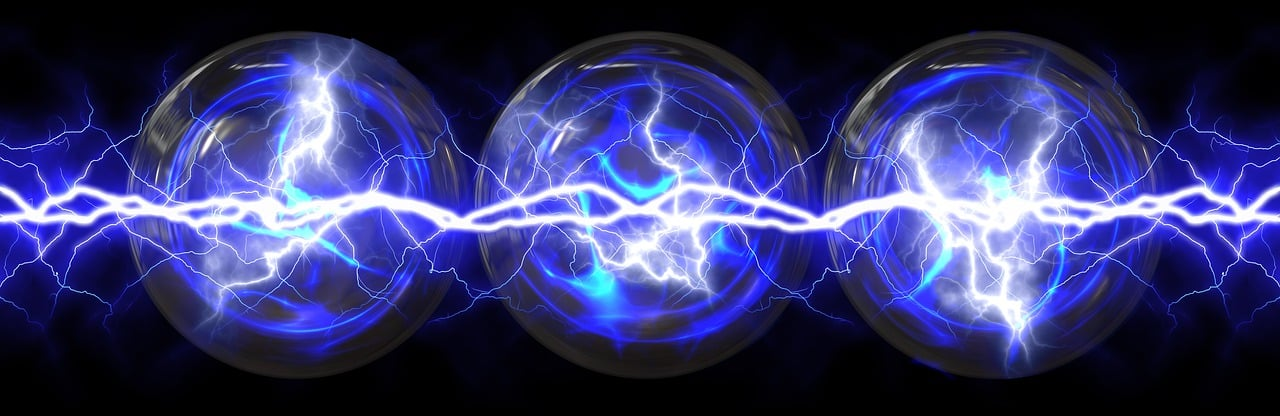# What Is Charge In Physics Class 10?

Electric charge is the physical property that makes it generate a force near other electrically charged objects. Like any other form of energy, the amount of charge that an object contains will vary.

The charge is usually measured in coulombs or electrons and can be negative or positive. An object with a negative electric charge is called an electron, and an object with a positive electric charge is called a proton.

The electric force between two charges occurs because poles repel each other while opposite poles attract one another.

The magnitude of the electric force between the charged objects depends on several factors, including the distance between their charges and their type of charge.

If two objects have charges of the same type, then doubling their separation distance will cause the magnitudes of their electric fields to reduce to 1/4.

The strength of an electric field also decreases with an increase in distance from a point charge. This is why static electricity is only found very near charged objects.## Is Electric Charge a Vector Quantity?

The quantity of electric charge is a scalar. In addition to size and direction, for a quantity to be considered a vector, it must also adhere to the rules of vector addition, such as the triangle law of vector addition and the parallelogram law of vector addition.

When two currents meet at a point in the instance of an electric current, the resultant current will be an arithmetic average and not a vector sum. Even though an electric current has magnitude and direction, it is a scalar quantity.

View Also :

What Is The Full Form Of Science?

Who Coined The Term Biology?

What Does ATM Stand For In Chemistry?

What Is Focus In Physics?

Who Is The Mother Of Indian Environmental Science?